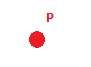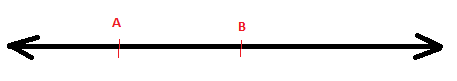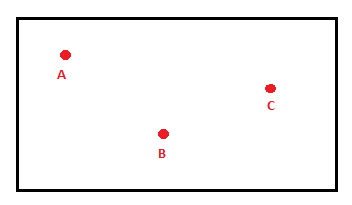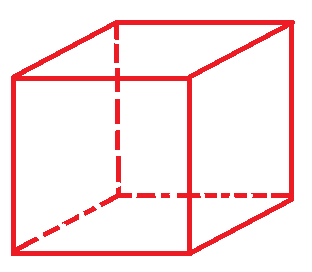# An introduction to geometry

A point in geometry is a location. It has no size i.e. no width, no length and no depth. A point is shown by a dot.A line is defined as a line of points that extends infinitely in two directions. It has one dimension, length. Points that are on the same line are called collinear points.A line is defined by two points and is written as shown below with an arrowhead.

$\\ \overset{\leftrightarrow}{AB } \\$

Two lines that meet in a point are called intersecting lines.

A part of a line that has defined endpoints is called a line segment.  A line segment as the segment between A and B above is written as:

$\overline{AB}$

A plane extends infinitely in two dimensions. It has no thickness. An example of a plane is a coordinate plane. A plane is named by three points in the plane that are not on the same line. Here below we see the plane ABC.A space extends infinitely in all directions and is a set of all points in three dimensions. You can think of a space as the inside of a box.## Video lesson

Plot a point, a line, a line segment and an angle in a coordinate plane.